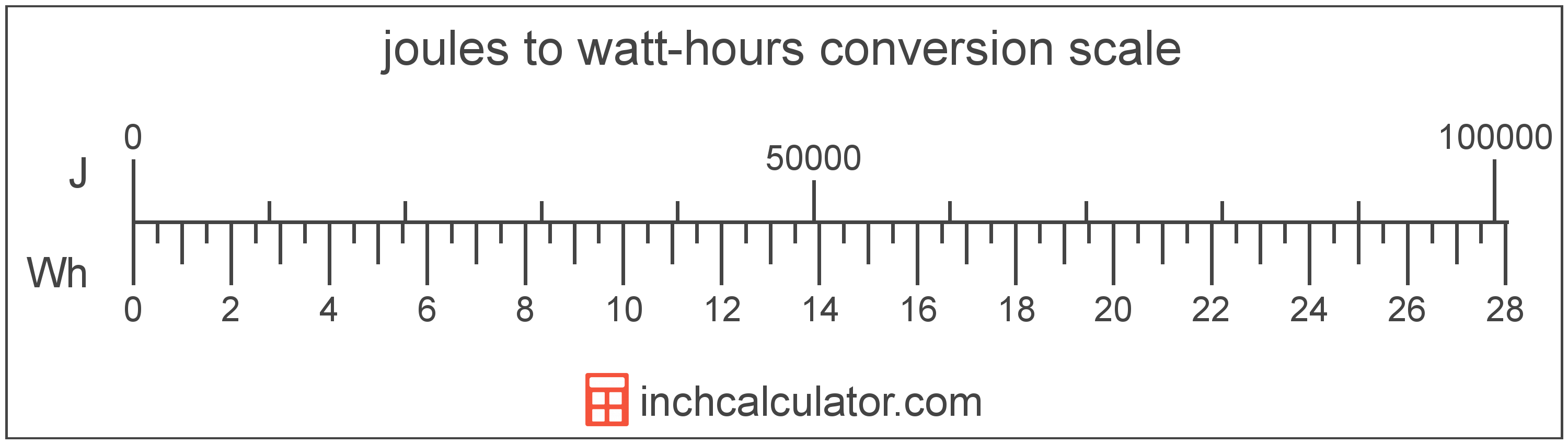# Joules to Watt-Hours Converter

Enter the energy in joules below to get the value converted to watt-hours.

Results in Watt-Hours:1 J = 0.000278 Wh

Do you want to convert watt-hours to joules?

## How to Convert Joules to Watt-Hours

To convert a measurement in joules to a measurement in watt-hours, divide the energy by the following conversion ratio: 3,600 joules/watt-hour.

Since one watt-hour is equal to 3,600 joules, you can use this simple formula to convert:

watt-hours = joules ÷ 3,600

The energy in watt-hours is equal to the energy in joules divided by 3,600.

For example, here's how to convert 5,000 joules to watt-hours using the formula above.
watt-hours = (5,000 J ÷ 3,600) = 1.388889 Wh## What Is a Joule?

The joule is the energy equal to the force on an object of one newton at a distance of one meter. One joule is equal to the heat energy dissipated by the current of one ampere through one ohm of resistance for one second.

One joule is also equal to the energy needed to move an electric charge of one coulomb through a potential difference of one volt. In addition, one joule is also equal to the one watt-second.

The joule is the SI derived unit for energy in the metric system. Joules can be abbreviated as J; for example, 1 joule can be written as 1 J.

## What Is a Watt-Hour?

The watt-hour is a measure of electrical energy equal to one watt of power over a one hour period.

Watt-hours are usually abbreviated as Wh, although the formally adopted expression is W·h. The abbreviation W h is also sometimes used. For example, 1 watt-hour can be written as 1 Wh, 1 W·h, or 1 W h.

In formal expressions, the centered dot (·) or space is used to separate units used to indicate multiplication in an expression and to avoid conflicting prefixes being misinterpreted as a unit symbol.

## Joule to Watt-Hour Conversion Table

Table showing various joule measurements converted to watt-hours.
Joules Watt-hours
1 J 0.000278 Wh
2 J 0.000556 Wh
3 J 0.000833 Wh
4 J 0.001111 Wh
5 J 0.001389 Wh
6 J 0.001667 Wh
7 J 0.001944 Wh
8 J 0.002222 Wh
9 J 0.0025 Wh
10 J 0.002778 Wh
20 J 0.005556 Wh
30 J 0.008333 Wh
40 J 0.011111 Wh
50 J 0.013889 Wh
60 J 0.016667 Wh
70 J 0.019444 Wh
80 J 0.022222 Wh
90 J 0.025 Wh
100 J 0.027778 Wh
200 J 0.055556 Wh
300 J 0.083333 Wh
400 J 0.111111 Wh
500 J 0.138889 Wh
600 J 0.166667 Wh
700 J 0.194444 Wh
800 J 0.222222 Wh
900 J 0.25 Wh
1,000 J 0.277778 Wh
2,000 J 0.555556 Wh
3,000 J 0.833333 Wh
4,000 J 1.1111 Wh
5,000 J 1.3889 Wh
6,000 J 1.6667 Wh
7,000 J 1.9444 Wh
8,000 J 2.2222 Wh
9,000 J 2.5 Wh
10,000 J 2.7778 Wh

## References

1. International Bureau of Weights and Measures, The International System of Units, 9th Edition, 2019, https://www.bipm.org/documents/20126/41483022/SI-Brochure-9-EN.pdf
2. Bureau International des Poids et Mesures, The International System of Units (SI), 9th edition, 2019, https://www.bipm.org/documents/20126/41483022/SI-Brochure-9-EN.pdf